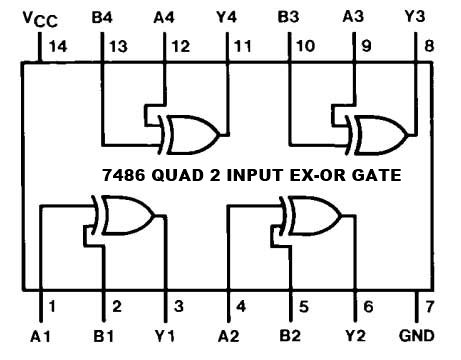# Exclusive OR gate (EXOR GATE) or XOR Gate

The output of an exclusive OR gate is HIGH if its one input, but not both is HIGH. The Output of EX-OR gate is HIGH if Inputs are different (one is high and another is low) and output is LOW when both input is same (Either low or high). The Exclusive OR gate is also called as EX-OR gate.

The name exclusive-OR gate is derived from the fact that the output of its is 1, only when exclusively one of its input is 1. The operation of the Exclusive –OR gate is denoted by Ꚛ. And the expression for the output of its circuit is given as  Z =A Ꚛ B =

$\overline{A}B+A\overline{B}$

(A bar.B Plus A .B bar)

### The circuit of EXOR (XOR) gate### The Equivalent circuit and Symbol of EXOR (XOR) gate### The truth table of EX-OR gateThe EX-Or gate has only two inputs, there are no 3 or 4 input EX-or gates. The operation of EX-OR gate is denoted by Ꚛ (plus sign surrounded by a circle).

The circuit of the EX-OR gate is also called an inequality comparator or detector because it produces output only when the two inputs are different. It means Output is 1 when inputs are not equal. It is also called anti-coincidence gate. It should be noted that the same Ex-OR Truth table applies when adding two binary digits(bits). The main property of an EX-OR gate is that can perform modulo-2 addition. A 2-input EX-OR circuit is sometimes called a modulo-2 adder or a half adder because the possible carry bit has not been taken, resulting from an addition of two preceding bits.

Three or more variable EX-OR gates do not exist. When more than two variables are to be Ex-ORed, a number of two input EX-OR gates will be used. The X-OR of a number of variables assumes a1 state only when an odd number of input variables assumes a 1 state.

# X-OR gate as an inverter

An EX-OR gate can be used as an inverter by connecting one of its two input terminals to logic 1 and feeding the input sequence to be inverted to the other terminal.

If the input bit is 0, the output is, 0 Ꚛ 1 = 1 and if the input bit is 1, the output is 1 Ꚛ 1= 0. So we can say that the Ex-OR gate can be used as a controlled inverter. Where one of the inputs can be used to decide whether the signal at the other input signal will be inverted or not.

The ic 7486 is a TTL packaging of EX-OR gates. And the 74C86 is a CMOS IC package both IC contains four EX-OR gates. High-speed CMOS IC 74HC86 contains four EX-Or gates.# Exclusive NOR gate

Exclusive NOR gate is also called as EX-NOR or X-NOR gate. An X-NOR gate is a combination of an X-OR gate and a NOT gate. This circuit operates just opposite to the X-OR circuit. The 4 output of this gates is HIGH if and only if its both inputs are same (either 0 or 1), and its output is LOW if inputs are different. It is also called coincidence gate because its output is 1 only when its input coincides (0, 0 or 1, 1). It can be used as an equality detector because its output is 1 only when its inputs are equal.

### Circuit Of EX-NOR (XNOR) gate### Symbol and Equivalent circuit of XNOR gateThree or more variable X-NOR gates do not exist. When a number of variables are to be X-NORed, a number of two-input X-NOR gates can be used. The X-NOR of a number of variables assumes a 1 state, only when an even number (including zero) of input variables assumes a 0 state.

### EXNOR (XNOR) Gate truth table# Ex-NOR gate as an inverter

An X-Nor gate can be used as an inverter by connecting one of two input terminals to logic 0 and feeding the input sequence to be inverted to the other terminal. If the input bit is a 1, the output is. If the input bit is 0, the output is. The X-NOR of two variables A and B is the complement of the X-OR of the two variables.

z = A Ꚛ B =

$AB+\overline{A}\overline{B}$

(A. B plus A bar.B bar)

The X-NOR gate can be used as a controlled inverter, that is, one of its inputs can be used to decide whether the signal at the other inputs will be inverted or not. The x-NOR gate can be used as a too.

But the X-NOR of three variables A, B, and C is not equal to the complement of the X-OR of A, Band C.

The X-Nor of a number of variables is equal to the complement of the X-OR of those variables only when the number of variables contains even.

The TTL IC 74LS266, the CMOS IC 74V266 and the high-speed CMOS IC 74HC266 is the packaging of four X-Nor gates in each.